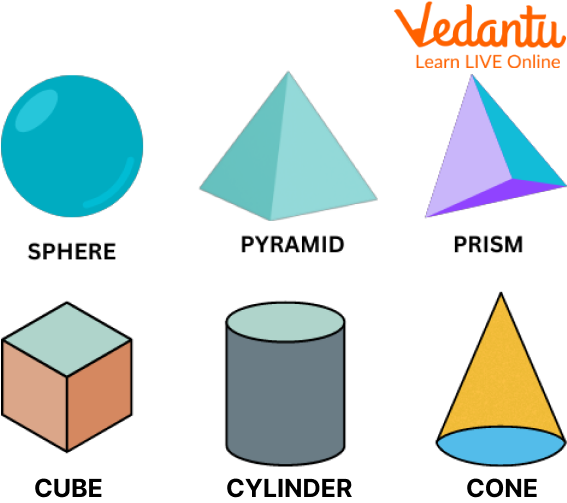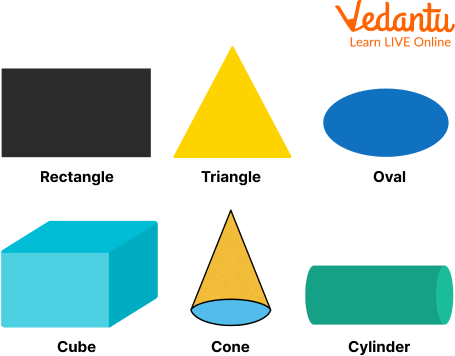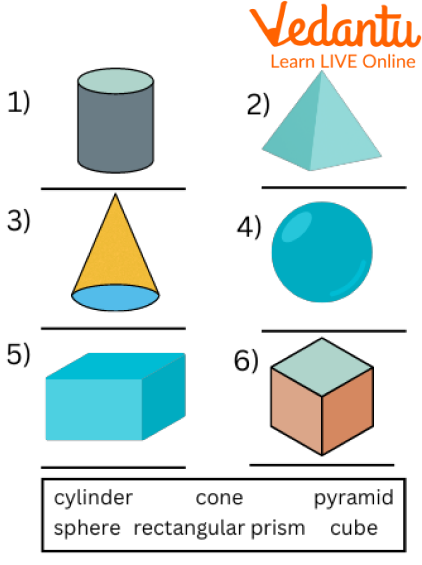Courses
Courses for Kids
Free study material
Free LIVE classes
More

# Comparing and Contrasting Three Dimensional FiguresLIVE
Join Vedantu’s FREE Mastercalss

## Learning Three-Dimensional Figures for Kids

You must have seen the ball at home with which you play during your free time. Ever wondered what shape it is? And what is it called? 3D shapes are solids or materials with three dimensions (length, width, and height), unlike two-dimensional objects with only two dimensions (length and width).

Three-dimensional figures include a cube, a pyramid, and a sphere. This article will discuss three-dimensional shapes, comparing and contrasting three-dimensional figures, the difference between two-dimensional and three-dimensional for kids, examples of 2d and 3d figures, and comparing 3d shapes worksheets.Different 3-dimensional Figures

## What are 2d and 3d Shapes?

3D shapes are solids with three dimensions: length, width (width), and height. The term 3D shapes refer to three-dimensional objects. A cube, for example, has all of its faces in the shape of a square.

Two-dimensional shapes have a length and a breadth. They have a surface area but no volume.

Triangles, squares, and rectangles are examples of 2D shapes.

## Examples of 2d and 3d Shapes

There are various types of 2 dimensional and 3-dimensional shapes used in Mathematics. The pictures of a few of these figures are given below, along with their respective names.## Difference Between Two-dimensional and Three-dimensional Figures

Knowing the difference between 2d and 3d shapes for kids can be quite easy once you know a few facts. Take a look at the differences between these two:

 2D- Shapes 3D-Shapes Two-dimensional figures are figures with a two-dimensional plane as their base. A three-dimensional figure is a figure with a three-dimensional plane as its base. A two-dimensional shape is a shape that is flat, meaning it does not exist in three dimensions. Examples of two-dimensional shapes are squares, triangles, and rectangles. A three-dimensional shape is a shape that is three-dimensional, meaning it exists in three dimensions. Examples of three-dimensional shapes are cubes, spheres, and pyramids. Two-dimensional shapes are shapes that have length and width but no height. For example, a square, rectangle, circle, or any other polygon. Three-dimensional shapes have height, length, and width. A cone is a three-dimensional shape because it has height, length, and width. A cube is also a three-dimensional shape because it has height, width, and length.

## Comparing 3d Shapes Worksheet: Solved Examples

Q1. Identify the 2D shapes from the following.

Circle, rectangular box, Rubik's cube, hexagon

Ans: Among the given shapes, a circle and a hexagon are 2D shapes because they do not have any thickness or depth. A rectangular box and a Rubik's cube are 3D shapes because they have 3 dimensions (length, width, and height).

Q2. 3D shapes have only length and width. Is this statement correct?

Ans: No, 3d shapes also have the dimension height along with length and width.

Q3. Solve the riddle.

(a) I only have 3 sides and 3 corners. Who am I?

Ans: I am a triangle.

(b) I have no sides and I am round. Who am I?

Ans: I am a circle.

## Practice Questions

Q1. Identify the solid shapes by choosing their names from the word box:Solid Shapes

Ans: 1. Cylinder

2. Pyramid

3. Cone

4. Sphere

5. Rectangular Prism

6. Cube

Q2. A circle has ___ sides and ____ corners.’

Ans: A circle has 0 sides and 0 corners.

Q3. I am a solid shape. I have one curved surface and no edges. Who am I?

Ans: Sphere

## Summary

A 2D shape is a flat shape that has only two dimensions – length and width, with no thickness or depth. That is the reason why it is called a two-dimensional shape. Later on, we came to know about the 3D shape. Compared to these, a 3D (three-dimensional) shape has three dimensions – length, width, and height. Then we saw the immediate difference between these two shapes. After that, we saw some solved examples and practice problems. After going through this article, do try at a practice problem.

Last updated date: 15th Sep 2023
Total views: 87.3k
Views today: 0.87k

## FAQs on Comparing and Contrasting Three Dimensional Figures

Q1. What are vertices in 3d shapes?

A vertex is a point where two or more lines intersect. In simple terms, it is referred to as a corner. The vertices are represented by the points where the edges intersect.

Q2. What are edges in 3d shapes?

A boundary edge is a line segment that connects one vertex (corner point) to another. They act as the meeting point of two faces.

Q3. What are faces in 3d shapes?

Any single flat or curving surface of a solid object is called a face.  3D geometries can have multiple faces.

Q4. What is the volume of a three-dimensional shape?

The volume of 3d Shapes is the amount of cubic space occupied by the shapes. We need the three dimensions measured to calculate the volume. Knowing the formula for each shape makes calculating the volume of 3D shapes easier.